Click to Chat

1800-1023-196

+91-120-4616500

CART 0

• 0

MY CART (5)

Use Coupon: CART20 and get 20% off on all online Study Material

ITEM
DETAILS
MRP
DISCOUNT
FINAL PRICE
Total Price: Rs.

There are no items in this cart.
Continue Shopping• Complete Physics Course - Class 11
• OFFERED PRICE: Rs. 2,968
• View Details

```Capacitors and Capacitance

Table of Content

What is Capacitor?

Capacitance in Physics

Construction of Capacitor

Role of Non-Conducting Dielectric in Capacitor

Capacitor Rating

Properties or Characteristics of Capacitor

Super Capacitor

Classification of Super Capacitors

Parallel Plate Capacitor

Capacity of Parallel Plate Capacitor

Effect of Dielectric on Capacitance of Capacitor

Energy Stored in a Capacitor

Numerical

What is Capacitor?

Capacitor also known as Electric-condenser is a two terminal electric components which has ability or capacity to store energy in the form of electric charge. Capacitors are usually designed to enhance and increase the effect of capacitance considering the capacitor’s sizeand shape. The storing capacity of capacitance vary from small storage to high storage.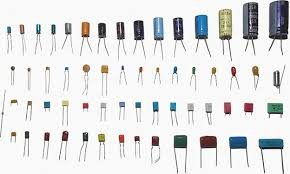Capacitors

Capacitance in Physics

The ability of an capacitor to store the energy in form of electric charge is known as Capacitance.

In other words capacitance can be described as the storing ability of a capacitor and it is measured in farads.

For Example, if we connected a capacitor to a 9 volt battery and measured that it stored 9 coulombs of charge, its capacitance would be 1 farad

Construction of Capacitor

Most capacitor usually contains two electrical conductors which are  surface separated by dielectric or between metallic plates.

Conductors may be in form of electrolyte, thin film, sintered bead of metal etc.Construction of Capacitor

Role of Non-Conducting Dielectric in Capacitor

The non conducting dielectric are used to increase capacity of capacitor to charge.Materials that are usually used as dielectrics in a capacitor are: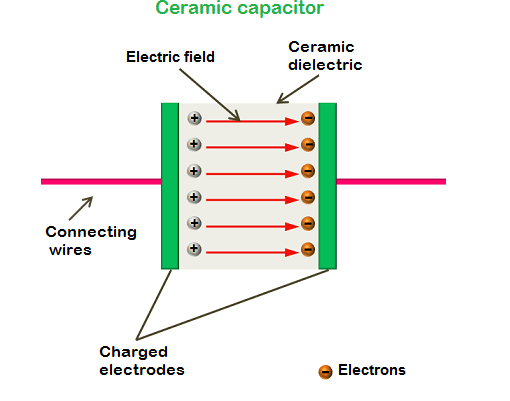Mica

Plastic film

Glass

Paper

Ceramic

Capacitors can be added in two ways

Either in Serial

Or in parallel

In Series

Capacitor C1 and C2 are connected in series. When the capacitors are connected serially then the total capacitance that is Ctotal is less any one of the capacitor’s capacitance.capacitors connected in series

The capacitance of capacitor connected in series can be calculated as: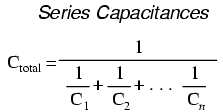In Parallel

Capacitor C1 and C2 are connected in parallel. When the capacitors are connected parallel then the total capacitance that is Ctotalis any one of the capacitor’s capacitance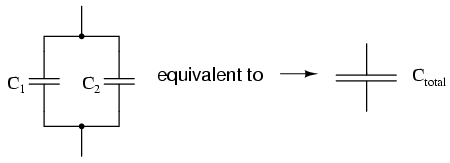Capacitor connected in parallel

The capacitance of capacitor connected in parallel can be calculated as:

Parallel Capacitances

Ctotal = C1 + C2 + …. Cn

Capacitor Rating

The capacitance value of two different capacitors may exactly be the same and the voltage rating of the two capacitors are different.

Let us take two capacitor one which has small voltage rating and other with high voltage rating then if a smaller rated voltage capacitor is substituted in place of a higher rated voltage capacitor, the increased voltage may damage the smaller capacitor.

The common working DC voltage of capacitor are printed on the capacitor and it be 10V, 16V, 25V, 35V, 50V, 63V, 100V, 160V, 250V, 400V and 1000V.Fig 1.5 DC voltage rating on Capacitor

Properties or Characteristics of Capacitor

The properties or characteristics of capacitors may differ from one another. Few characteristics of capacitors are:

Nominal Capacitance, ( C ): This property of capacitor is important of all the properties of capacitors. This is measured in pico-Farads (pF), nano-Farads (nF) or micro-Farads (µF) and is printed on capacitor body in form of number or text.

Working Voltage, ( WV ): The total amount of direct current (DC) or alternating current (AC) is applied to a capacitor without any failure in the capacitor’s whole lifetime. This above stated amount can be defined through working voltage.

Tolerance, ( ±% ): Just like voltage rating, capacitor also have tolerance rating which vary from plus to minus value either in picofarad’s (±pF) for low value capacitors generally less than 100pF or as a percentage (±%) for higher value capacitors generally higher than 100pF.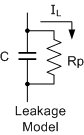Leakage Current: The capacitors Leakage Current is the small DC current flow in the region of Nano-Amps (nA). Leakage current is a result of electrons physically making their way through the dielectric medium, around its edges or across its leads and which will over time fully discharging the capacitor if the supply voltage is removed.

Super Capacitor

Super Capacitor also known as Electric Double-Layer Capacitor (EDLC), Supercap, Ultracapacitor or Goldcap has the capacitance value much higher as compared to other Capacitors but with lower voltage limits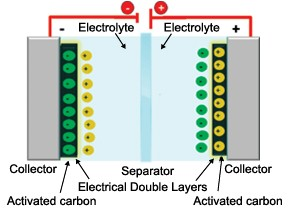Classification of Super Capacitors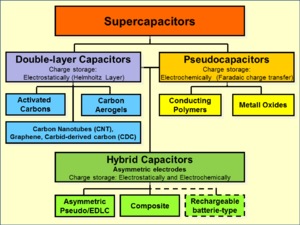Parallel Plate Capacitor

The setup of dielectric and electrode to form a capacitor is known as parallel plate capacitor.

The two parallel conductive plates (electrodes) are used and in between medium of dielectric. The value of capacitance is dependent on the material or the medium between the two plates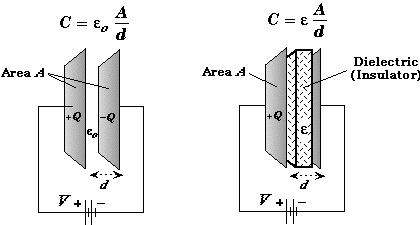A is the common area of the two plates and d is the distance between the plates.

Capacity of Parallel Plate Capacitor

Cair = ε0A/d

Cmed = Kε0A/d

Here, A is the common area of the two plates and d is the distance between the plates.

Effect of Dielectric on Capacitance of Capacitor

Faraday have given this theory, that is if the space between two electrodes are filled with dielectric the capacitance of the capacitor will be increased.

If all the space is filled by a dielectric that is there is no place left then the capacitance of a capacitor will be increased by K. and here K is known as Dielectric Constant.Effect of Dielectric on Capacitor

Proof

Consider the following diagramDielectric between two capacitors

E = Eo – Ep
The electric field Eo in the outside region of the dielectric will be null. Now the equation of the potential difference between two plates is

V =o (d-t) + Et
But Eo= Er or K

Therefore E= Eo / k

So

V = E o (d-t) + Eot / k

V = Eo [d-t+t/k]

As we know

Eo =  σ/ε0
= Q / A ε0

V = Q / A ε0 [d – t + t/k]

Capacitance of capacitor is:

C = Q/V

= A ε0 /(d – t + t/k)
= A /d – t (1-1/K)

that is, C=   A/ d-t (1-1/k)

So,C > Co
Clearly, it is proved that if a dielectric slab is placed in the plates of a capacitor then its capacitance will increase by some amount.

Energy Stored in a Capacitor

The work done on moving a positive charge from a negative conductor to a positive conductor against the repulsive forces is known as Energy Stored in Capacitor.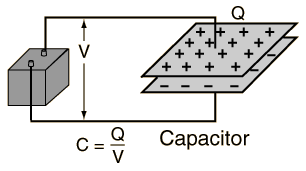It can be calculated as: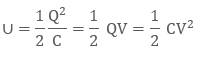Numerical

Given below a diagram of capacitors connected serially.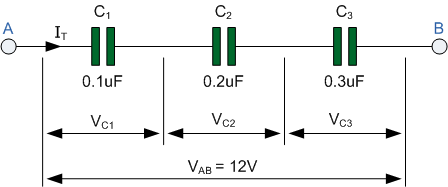Calculate the total capacitance?

Taking the three capacitor values, we can calculate the total capacitance, CT for the three capacitors in series as: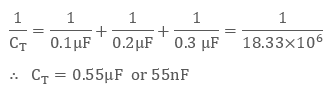Calculate the combined capacitance in micro-Farads (uF) of the following capacitors when they are connected together in a parallel combination:

two capacitors each with a capacitance of 47nF

one capacitor of 470nF connected in parallel to a capacitor of 1uF

Total Capacitance,

CT = C1 + C2 = 47nF + 47nF = 94nF or 0.094uF

Total Capacitance,

CT = C1 + C2 = 470nF + 1uF

therefore, CT = 470nF + 1000nF = 1470nF or 1.47uF

So, the total or equivalent capacitance, CT of an electrical circuit containing two or more Capacitors in Parallel is the sum of the all the individual capacitance’s added together as the effective area of the plates is increased.

(Related to parallel plate capacitor)

A parallel plate capacitor consists of two metal plates, each of area A = 150 cm2, separated by a vacuum gap d = 0.60 cm thick. What is the capacitance of this device? What potential difference must be applied between the plates if the capacitor is to hold a charge of magnitude Q = 1.00 ×10-3 µC  on each plate?

The capacitance   is given byThe voltage difference between the plates and the magnitude of the charge Q stored on each plate are related via, C = Q/V.

Hence, if Q = 1.00 X 10-3

then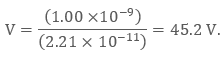(Related to energy stored in capacitor)

An air-filled parallel plate capacitor has a capacitance of  pF. A potential of 100V is applied across the plates, which are  cm apart, using a storage battery. What is the energy stored in the capacitor? Suppose that the battery is disconnected, and the plates are moved until they are  cm apart. What now is the energy stored in the capacitor? Suppose, instead, that the battery is left connected, and the plates are again moved until they are  cm apart. What is the energy stored in the capacitor in this case?

​The initial energy stored in the capacitor isWhen the spacing between the plates is doubled, the capacitance of the capacitor is halved to pF. If the battery is disconnected then this process takes place at constant charge Q. Thus, it follows from the formulathat the energy stored in the capacitor doubles. So, the new energy is 5.0 x 10-8 J. Incidentally, the increased energy of the capacitor is accounted for by the work done in pulling the capacitor plates apart (since these plates are oppositely charged, they attract one another.

If the battery is left connected, then the capacitance is still halved, but now the process takes place at constant voltage V. It follows from the formulathat the energy stored in the capacitor is halved. So, the new energy is 1.25 x 10-8J. Incidentally, the energy lost by the capacitor is given to the battery (in effect, it goes to re-charging the battery). Likewise, the work done in pulling the plates apart is also given to the battery.

Capacitors and Capacitance
```### Course Features

• 101 Video Lectures
• Revision Notes
• Previous Year Papers
• Mind Map
• Study Planner
• NCERT Solutions
• Discussion Forum
• Test paper with Video Solution